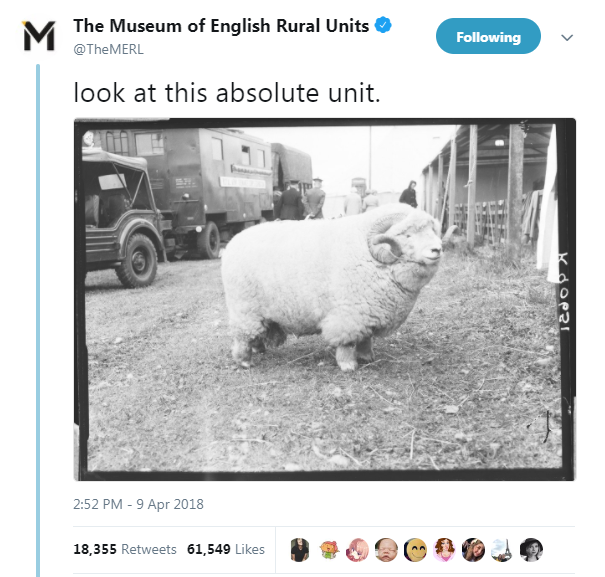Christian Lawson-Perfect, Newcastle University

Motivation

Numbas has been used mainly for pure maths.

Every now and then, someone would ask to mark a quantity with units, and I'd be like

🤷

and then

“It's on my to-do list”

Motivation

Recently, Nick Walker asked for units, which are very important in chemistry.

What we wanted

• Student gives a number and its units.
• It's marked correct if they give the right units.
(and the right number)
• Helpful feedback if they use the wrong units.

NOT bothered about algebraic expressions involving units

So we needed

• A big list of units.
• Conversion factors between units.
• Code to parse expressions like
88 cm^2/J*s.

Down the rabbit hole

GNU units knows just about everything ever.

But there's no JavaScript implementation.

Either
write code to parse its source file myself,
or
find a less-perfect option.

I was in a hurry, OK?

js-quantities does a decent job:

• Knows most common units.
• Parses units expressions.
• Converts between units.
• "Are two units compatible" check.

Implementation

From there, it was very easy:

• Add a quantity data type to JME.
• Define JME functions wrapping js-quantities.
• Make a custom part type marking "quantity with units".
• Explore the possibilities!

Minus

Both js-quantities and Numbas use JS' built-in floating point numbers: only ~15 d.p. of accuracy.

Trouble with numbers like Avogadro's constant: $$6.02214086 \times 10^{23} \textrm{mol}^{-1}$$.

Using more precise numbers would involve modifying js-quantities.

Now on my to-do list! ✔️

Plus

Very easy to use:

width = quantity(random(20..50#5), "cm")

length = quantity(random(50..250#10),"cm") as random("m","cm")

height = quantity(random(10..scalar(width as "cm")#10),"cm")

volume = width*length*height as "m^3"